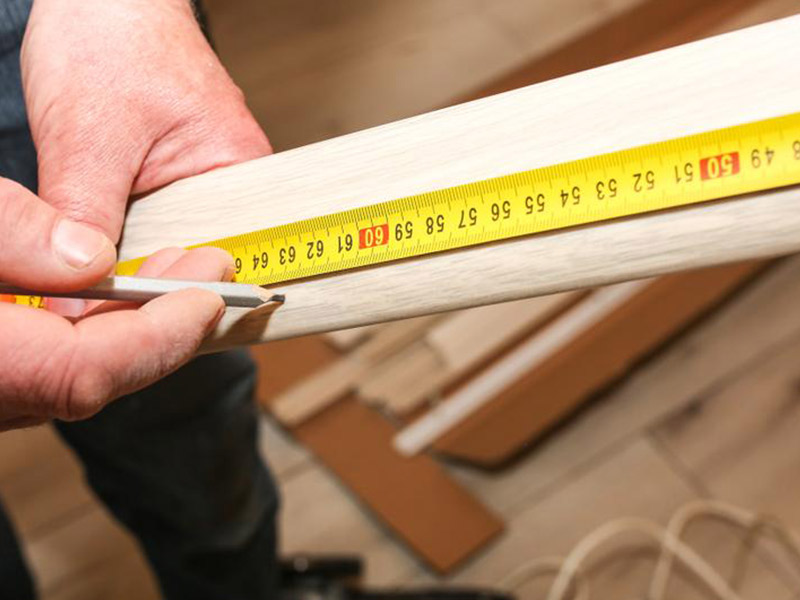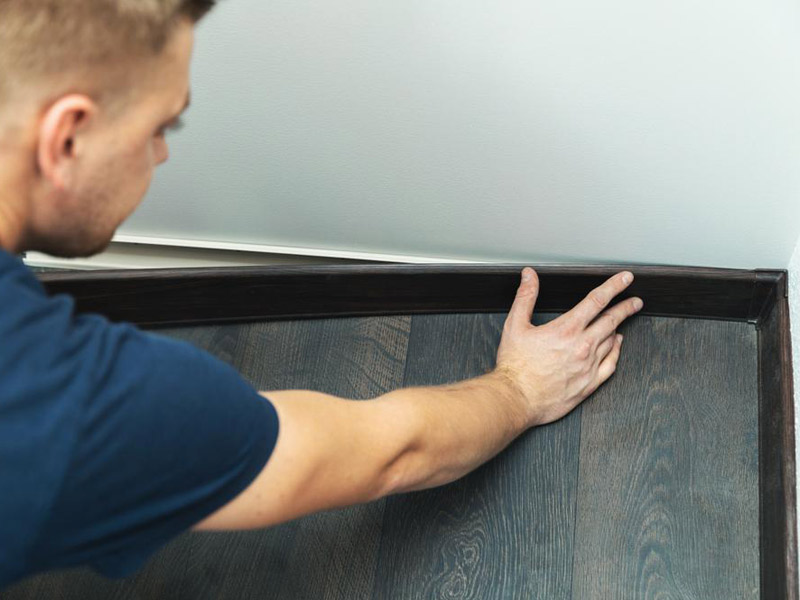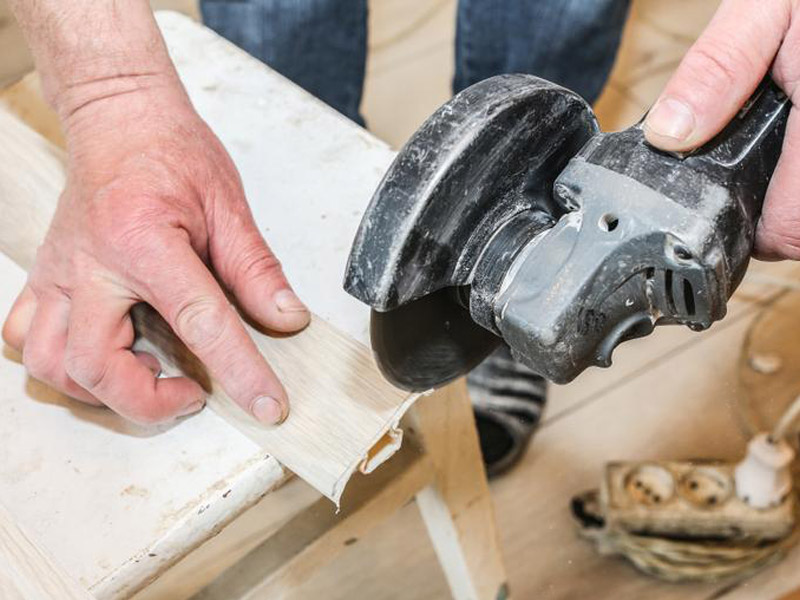# How Much Baseboard Do I Need for My House?

Written by Milan Jara on 7th Oct 2022How much baseboard do I need? The math may be tricky, but we give you the formula and show you how to calculate how much you’ll need.

For those confused between square footage and linear foot measurement, there is an easy way to calculate and differentiate between the two. Both have different applications within the home; if you don’t use the proper calculation when buying material, you may not have enough material to finish the job. We will teach you to figure out how much baseboard you need for your home, whether you’re using an MDF baseboard, wood baseboard, or even when installing an electric baseboard or baseboard heating system.

The term linear means “along a straight line.” It is a length measurement needed when calculating measurements for any interior trim, doors and window casing, baseboard trim, electric baseboard heaters, countertops, and walls. You can use it for anything with a straight line.

### Calculating Linear FeetCalculate linear footage in a room by measuring length times width. Once you have these measurements, multiply each of the numbers by two, then add them together. An example for a 10’ x 12’ room would be:

Length times two plus width times two
(10 x 2) + (12 x 2) = 44 linear feet.

If you measure in inches, then you will need to do the following:

Stretch your tape measure tightly from one end of a wall to the other in a straight line. Then, write down the length of the wall in inches and multiply it by two. Do the same for the width. After calculating this, add them together for linear feet as outlined above.

Then, take this number, and divide it by twelve to convert your inches to linear feet.

Here’s an example. If we take the above dimensions and convert them into inches, it would be 120” x 144”. To calculate linear feet (converting inches into feet), you would do it in the following way:

Length times two plus width times two
(120” x 2) + (144” x 2) = 528 square inches

Then, we divide by 12 (12 inches equals one foot) to calculate linear feet.
528” / 12 = 44 linear feet

You will need to account for overage, which would be 10 to 20%, to account for any waste, offcuts, overlapping joints, or damages that may occur when installing baseboards in your house.

### How Much Trim Should I Buy?

To calculate the amount of trim required for your entire house, you will need to calculate each room individually to obtain your total. Be sure to subtract the length of any doors or entrances that break up the room from the perimeter measurement.

You will still need to add 10% for overages. Even if you don’t use them, they are handy to have around in case there is any damage or a piece needs replacing.

To sum it up, to calculate for your entire home, you will need to do the following steps:

1. Calculate the wall length of each room in linear feet or inches.
2. Subtract the measurement of any entrances, closets, and doors.
3. If you calculated in inches, convert to linear feet.
4. Do this for each room in the house.
5. Add the total in linear feet together.
6. Add a minimum of 10% for overages.
7. Now, you have your total linear footage indicating how many baseboards you will need to purchase.

Calculate for baseboards using linear square feet. If math isn’t your thing, you can use a baseboard calculator.### Are Baseboards Worth Replacing or Installing?

Most people would answer yes to this question. Baseboards hide noticeable seams where the wall meets the floor to give a finished appearance. If the old baseboard trim shows signs of aging, paint is one option. However, it is crucial to first prep the area, which can take more time than installing new ones.

Replacement is always better for stained, warped, or water-damaged boards.

### Should Baseboards Be Glued or Nailed?

You can install solid wood baseboard or MDF baseboard using a combination of adhesive and finish nails. This gives a tight connection between the baseboards and the wall. Adhesive will prevent your MDF or solid wood baseboard from bowing as rooms may shift between wall studs. Nails will keep it locked into position when the glue sets and provide an added layer of security.

### What Types of Joints Are Used When Installing Baseboard?You can use three types of joints when installing baseboard trim. These are:

• Scarf Joints: This makes two shorter boards appear as a single long piece. You start by making an overlapping 45° angle on the ends of two boards. When installing them, marry the angles, and the joints will disappear. Be sure you nail through the joint for the best results.
• Miter Joint: You can form outside corners with a 45° cut at the end of two boards. They can fit perfectly together using a 90° angle where the walls meet. If you are cutting an inside angle, you can do it using the same method. However, professionals recommend a coping joint.
• Coped Joint: Use these for inside corners. After cutting them at a 45° angle, you follow your molding profile closely with a coping saw. The saw will need to be held at the proper angle so that you can slightly back-cut the material, resulting in a precise pattern that will easily fit over the opposite trim and make the perfect inside corner.

If any gaps occur, you can quickly fix them using the methods outlined here.

### Conclusion

If you want to know how much baseboard you need for your home, you will need to calculate the linear footage of each room, minus closets, doors, etc., and add the perimeter of each room together. Then, add an additional 10% for overages to get your total. While the math may appear extensive, it will provide you with the most accurate measurement.